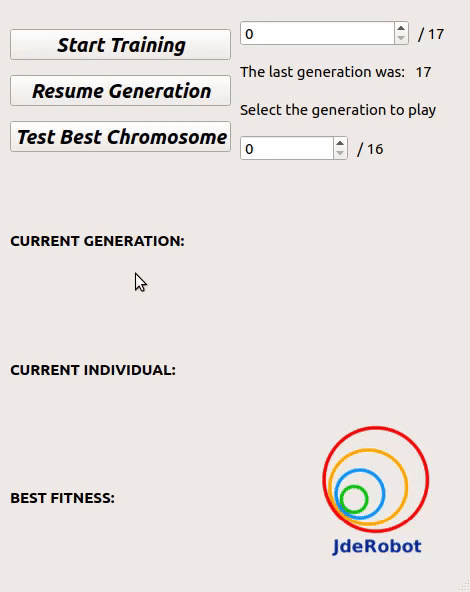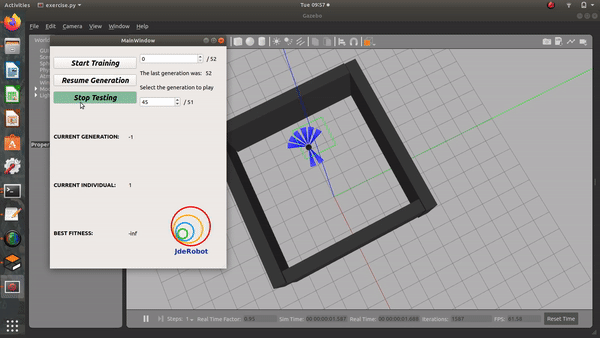Tuning the parameters of the Gazebo simulation and the MyAlgorithm specifics was done in this week.

## Where does this fit in the project?

The first problem of the exercise, was that the training was still a little slow. It was due to the different running speeds of the algorithm and the Gazebo simulator. A little tweaking with the physics engine update rate, this problem was solved. However, even after obtaining the perfect exercise, the exercise solution is completely random. Consistent results are not obtained after training. Sometimes, the robot learns a perfect obstacle avoidance behaviour. However, sometimes, just goes straight and collides with the wall.

This should not be expected from an exercise that students should be solving. Having random results, wouldn’t be preffered. Therefore, improving on this problem is a very crucial thing to do!

## Outcome of this WeekThis was the best combination of training speed and learningThe training is very random, sometimes the robot learns and sometimes it does not

The gifs show pretty much all of the outcome.

## Logic of the Code

On suggestion of Luis Sir, I checked the update rate of the Gazebo simulation. Gazebo works on a physics engine that renders the dynamics of the simulation. By default, Gazebo runs using the physics engine ODE(Open Dynamics Engine). The parameters of our interest are:

• `max_step_size`: The maximum time step size that can be taken by a variable time-step solver (such as simbody) during simulation. For physics engines with fixed-step solvers (like ODE), this is simply the time step size. The default value in Gazebo is `0.001` seconds

• `real_time_factor`: `max_step_size x real_time_update_rate` sets an upper bound of `real_time_factor`. If `real_time_factor < 1` the simulation is slower than real time.

• `real_time_update_rate`: This is the frequency at which the simulation time steps are advanced. The default value in Gazebo is `1000 Hz`. Multiplying with the default `max_step_size` of `0.001` seconds gives a `real_time_factor` of `1`. If `real_time_update_rate` is set to `0` the simulation will run as fast as it can. If Gazebo is not able to update at the desired rate, it will update as fast as it can, based on the computing power.